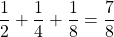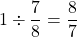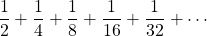# Anatomy of a Puzzle

Recently I was asked to provide a Puzzle For Today for the BBC Radio 4 Today programme which was partially coming as an Outside Broadcast from Ulster University.

I’ve written a post about the puzzle itself, and some of the ramifications of it; this post is really more about the thought process that went into constructing it.

When I was first asked to do this I had a look at the #PuzzleForToday hashtag on Twitter and found that a lot of people found the puzzles pretty hard, so I thought I might try to construct a two part puzzle with one part being relatively easy and the other a bit harder. I also wanted something relatively easy to remember and work out in your head for those people driving, since commuters probably make up a lot of the Today programme audience.

A lot of my students will know I often do puzzles with them in class, but most are quite visual, or are really very classic puzzles, so I needed something else. Trying to find something topical I thought about setting a puzzle around a possible second referendum election, since this was much in the news at the time. My first go at this was coming up with a scenario about an election count, and different ballot counters with different speeds counting a lot of ballots.

I constructed an idea that a Returning Officer had a ballot with a team of people to count votes. One of the team could count all the votes in two hours, the next in four hours, and the next in eight hours. But how long would they take if they worked all together? The second part would be: if there were more counters available but each took twice as long again as the one before, what was the least possible time to complete the task.

I liked this idea because I thought there were a lot of formal and informal ways to get to the answer, and indeed the answers I saw on Twitter and Facebook confirmed this. Perhaps the easiest way to approach the puzzle is this: to consider how much work each can do in an hour. We see the first person gets half of it done, the next one quarter and the next one eighth. All together then:We need to work out what number we would multiply by this to get one – i.e. the whole job being done. In this case it works out aswhich works out (a bit imprecisely) with a bit of work, or a decent calculator, as one hour, eight minutes and thirty four seconds and a bit. So, not great, but the second part of the puzzle works out much more smoothly. If we keep on with out pattern, we getFormally, this is called a Geometric Progression, there is a constant factor between each terms. Sometimes these infinite sums actually have a finite answer, which might be surprising. If you keep adding these fractions you might see that are adding ever closer to 1. Therefore this potentially infinite number of counters gets the work done in one hour, it can’t be less.

So, I was happy the second number worked out nicely, but the first is pretty tricky, and not easily worked out in one’s head. So I wondered what numbers I could use instead that would work out as an exact number of minutes. This really means that my three fractions from the first part of the problem, divide perfectly into 60. I used some Python to help me with this with a list comprehension:

# Establish a top size to work with n = 100
# Find all the triples where# a,b,c <= n and 1/a + 1/b + 1/c
# divides evenly into 60

triples = [(a,b,c) for a in range(1,n+1)
for b in range(1,a)
for c in range(1,b)
if (60/(1/a + 1/b + 1/c)).is_integer()]

It turns out that restricting ourselves to three numbers for the first quiz all of which are under 100 that there are 902 such sets of numbers. The very smallest numbers are 1, 2 and 6. The problem is that most of these triples don’t have the property of my original choices – which that there is a common number multiplied between the first and second, and the second and third etc.. That would make the second part of the puzzle more difficult.

So, I modified my list comprehension a bit to add the condition that there was a common ratio from a to b to c:

# Establish a top size to work with
n = 100

# Find all the triples where a,b,c <= n and 1/a + 1/b + 1/c
# divides evenly into 60 and where the factor between a and b,
# is the same as that between b and c

geometric_triples = [(a,b,c) for a in range(1,n+1)
for b in range(1,a)
for c in range(1,b)
if (60/(1/a + 1/b + 1/c)).is_integer()
and a/b == b/c]



This produced just three triples (with all numbers under 100):

>>> geometric_triples
[(28, 14, 7), (56, 28, 14), (84, 42, 21)]


and you can see these are all quite related. So I grabbed the first three numbers to try and keep the puzzle small.

As well as that, the programme team wanted a different focus than an election – they were a bit worried that because it was in the news so much it would be better to have another focus. I considered a computational task divided between processors, but eventually concluded this wouldn’t make a lot of sense to some listeners, so I went with this final configuration of the puzzle.

Part One

A Professor gives her team of three PhD students many calculations to perform. The first student is the most experienced and can complete the job on her own in 7 hours, the next would take 14 hours on his own, and the last would take 28 hours working single handed to complete the task. How long would the task take if they all worked together?

Part Two

If the Professor has more helpers, but which follow the same pattern of numbers to complete the task, what is the absolute minimum time the task can take?

You can probably answer this from the details of the construction above, but if not, you can always cheat here (the BBC programme page) or here.

# Establish a top size to work with n = 100
# Find all the triples where a,b,c <= n and 1/a + 1/b + 1/c
# divides evenly into 60 and where the factor between a and b,
# is the same as that between b and c
geometric_triples = [(a,b,c) for a in range(1,n+1) for b in range(1,a) for c in range(1,b) if (60/(1/a + 1/b + 1/c)).is_integer() and a/b == b/c]

Posted

in

by

Tags: